# 航空发动机部件老化对涡轮性能的影响研究Study of the Effect of Engine Component Deterioration on Turbine Performance

DOI: 10.12677/DSC.2019.84023, PDF, HTML, XML, 下载: 355  浏览: 534

Abstract: This paper focuses on the performance degradation of compressor and turbine core components. Considering three conditions: performance deterioration of compressor, turbine, compressor and turbine, embedding component performance deterioration model into stable engine computation model and modifying component characteristic, change of turbine component was analyzed. The matching turbine component model was established based on floating field simulating technology, the turbine floating field and the pressure field were calculated by modifying inlet and outlet boundary condition and rotor speed based on deterioration model, and the total performance was analyzed around performance deterioration. The results offer theoretical reference to single component even engine performance deterioration and effect between components.

1. 引言

2. 部件老化计算模型

$eta1=\left({\eta }^{\prime }-{\eta }_{0}\right)/{\eta }_{0}$ (1)

$eta2=\left({m}^{\prime }-{m}_{0}\right)/{m}_{0}$ (2)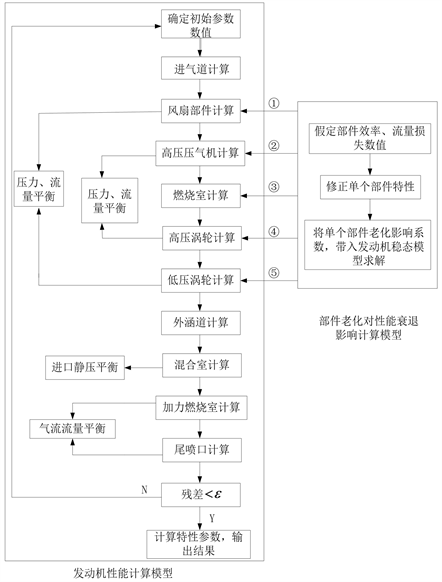Figure 1. Computation flow chart of effect of component deterioration on engine performance

3. 建立流场仿真模型

4. 老化模型与流场仿真混合求解

$\frac{\partial \left(\rho {u}_{i}\right)}{\partial {x}_{i}}=0$ (3)

$\frac{\partial \left(\rho {u}_{j}{u}_{i}\right)}{\partial {x}_{j}}=-\frac{\partial p}{\partial {x}_{j}}+\frac{\partial \left[{\mu }_{e}\left(\frac{\partial {u}_{i}}{\partial {x}_{j}}+\frac{\partial {u}_{j}}{\partial {x}_{i}}\right)\right]}{\partial {x}_{j}}$ (4)

“Spalart-Allmaras (S-A)”湍流模型方程如下：

$\frac{\partial }{\partial t}\left(\rho \stackrel{˜}{\upsilon }\right)+\frac{\partial }{\partial {x}_{i}}\left(\rho \stackrel{˜}{\upsilon }{u}_{i}\right)={G}_{\upsilon }+\frac{1}{{\sigma }_{\stackrel{˜}{v}}}\left[\frac{\partial }{\partial {x}_{j}}\left\{\left(\mu +\rho \stackrel{˜}{\upsilon }\right)\frac{\partial \stackrel{˜}{\upsilon }}{\partial {x}_{j}}\right\}+{C}_{b2}\rho {\left(\frac{\partial \stackrel{˜}{\upsilon }}{\partial {x}_{j}}\right)}^{2}\right]-{Y}_{v}+{S}_{\stackrel{˜}{v}}$ (5)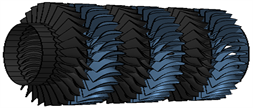(a)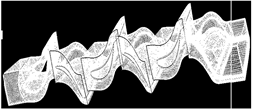(b)

Figure 2. Generated turbine model and grid of single passageTable 1. Boundary condition and rotor speedFigure 3. Inlet total temperature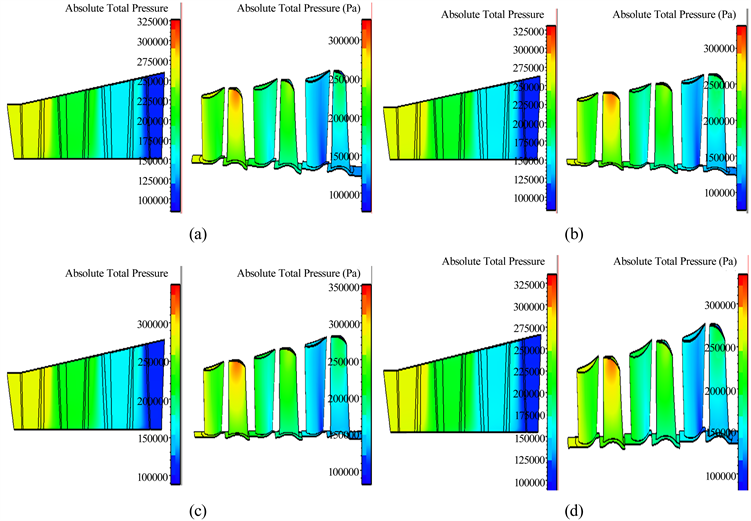Figure 4. Total pressure distribution in floating field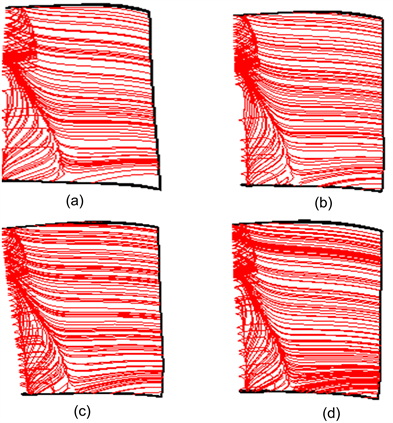Figure 5. Flow field distribution in rotor blasé of stage 1 (pressure surface)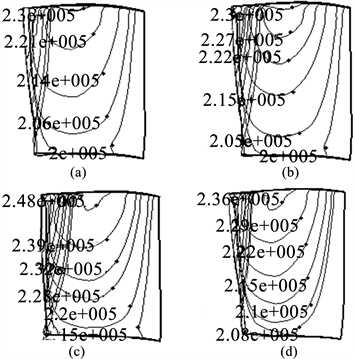Figure 6. Contour line distribution of static pressure in rotor blade of stage 1Figure 7. Total pressure distribution in 50% surface of turbine blade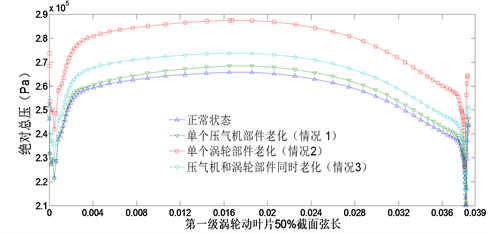Figure 8. Total pressure distribution in the chord of 50% surface of stage 1 rotor blade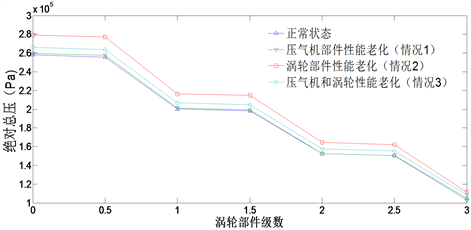Figure 9. Variation of turbine total pressure in the stage

5. 结论

1) 在稳态模型的基础上通过修改进出口边界条件以及转速进行流场仿真。得到两个部件同时老化对于涡轮性能的影响，并不是两个单独部件对涡轮的性能简单叠加，而是受制于各部件之间的相互制约，损失相对来说并不大。

2) 当单个部件或更多出现小幅度损失，而且这种损失还未使涡轮部件性能出现根本变化时，压力场、气流流场并不会出现较大程度的改变。气流分离提前，略靠近叶片前缘，等值压力线分布基本不变，但压力数值增加。

3) 压气机性能损失会使涡轮总温减小；而涡轮性能损失会使总温升高总压在叶片弦长中部变化较大，两端变化较小。对于多级涡轮来讲，在前面级的性能变化大，后面级的性能变化较小。

  于锦禄, 何立明, 孙冬, 等. 基于分形理论的航空发动机推力性能规律研究[J]. 航空动力学报, 2010, 25(10): 2302-2306.  李天亮. 航空发动机性能衰退规律研究及其诊断[D]: [博士学位论文]. 西安: 空军工程大学, 2008.  Balan, C. and Tabakoff, W. (1983) A Method of Predicting the Performance Deterioration of a Compressor Cascade Due to Sand Erosion. 21st Aerospace Sciences Meeting, 10-13 January 1983, 83-0178.  Kurz, R. and Brun, K. (2001) Degradation in Gas Turbine System. ASME Turbo Expo 2000: Power for Land, Sea, and Air, Munich, 8-11 May 2000, 2000-GT-0345. https://doi.org/10.1115/2000-GT-0345  Kurz, R. and Brun, K. (2001) Degradation in Gas Turbine System. Journal of En-gineering for Gas Turbines and Power, 123, 70-77. https://doi.org/10.1115/1.1340629  王占学, 刘增文, 叶新农. 某型涡扇发动机部件老化对性能影响的分析与计算[J]. 航空动力学报, 2007, 22(5): 792-796.  李本威, 李冬, 沈伟, 等. 涡轮叶片粗糙度对其性能衰退的影响研究[J]. 航空计算技术, 2009, 39(5): 26-29.  Clerc, M. and Kennedy, J. (2002) The Particle Swarm—Explosion, Stability, and Convergence in a Multidimensional Complex Space. IEEE Transactions on Evolutionary Compu-tation, 6, 58-73. https://doi.org/10.1109/4235.985692  周敏, 王如根. 改变开孔位置对风扇叶片气动特性的影响[J]. 汽轮机技术, 2007, 49(5): 359-361.  王如根, 周敏, 赵英武, 等. 跨声速压气机低雷诺数下流动失稳机制研究[J]. 航空动力学报, 2009, 24(2): 414-419.